# E-PolyLearning

 11. Which of the following is not a unit of mass transfer co-efficient ?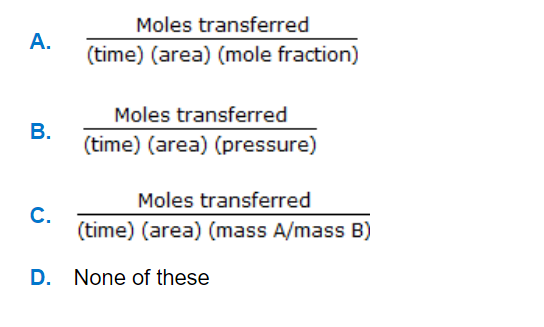a. A b. B c. C d. D

 12. The surface renewal frequency in Danckwerts' model of mass transfer is given by (KL: mass transfer co-efficient)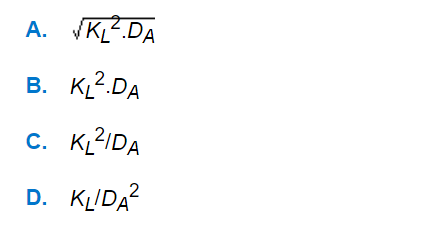a. A b. B c. C d. D
 13. A distillation column separates 10000 kg/hr of a benzene-toluene mixture as shown in the figure below. In the figure xF, xD and xW represent the weight fraction of benzene in the feed, distillate and residue respectively. The reflux ratio is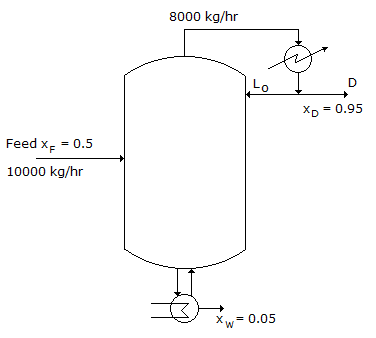a. 0.5 b. 0.6 c. 1.0 d. 2.0
 14. Which of the following is the correct ternery diagram for liquid-liquid extraction system comprising of two pairs partly soluble (e.g., chlorobenzene-water-methyl ethyl ketone system) ?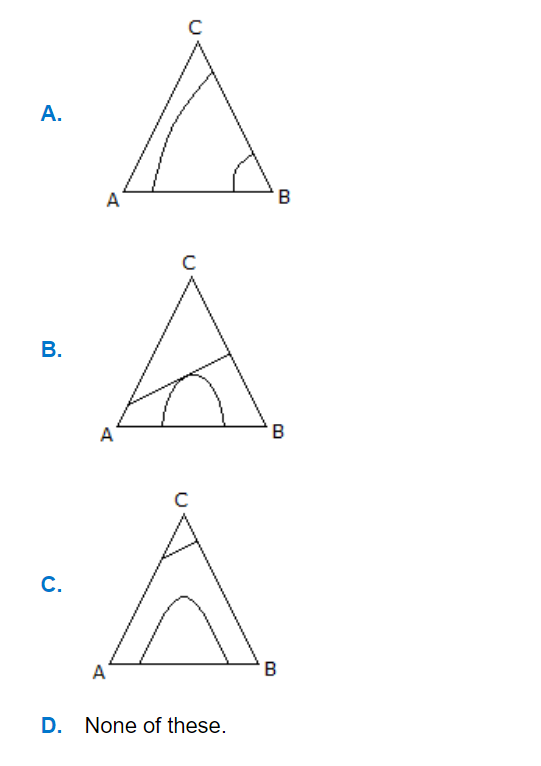a. A b. B c. C d. D
 15. If moisture content of solid on dry basis is X, then the same on wet basis is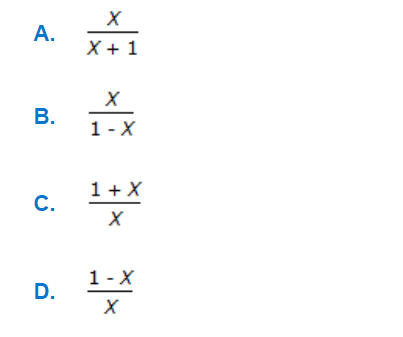a. A b. B c. C d. D